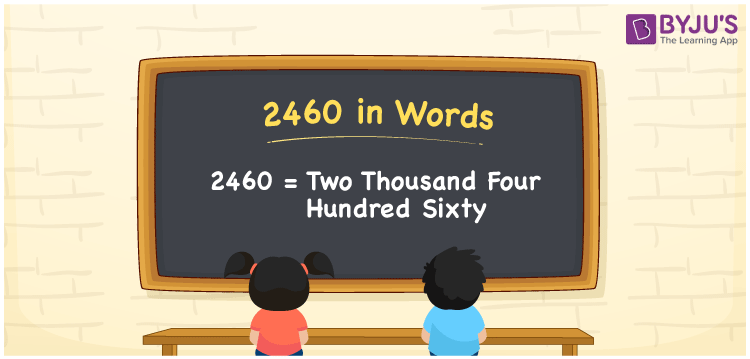# 2460 in Words

2460 in words is Two Thousand Four Hundred Sixty. The number 2460 is a cardinal number, as it is used to count things, measure distance, relate to money and so on. For example, we have to travel 2460 km to reach Rahul’s home. In this article, we are going to discuss the method of writing the number 2460 in words using the place value system.

 2460 in Words: Two Thousand Four Hundred Sixty. Two Thousand Four Hundred Sixty in Numerical Form: 2460.

## 2460 in English Words## How to Write 2460 in Words?

The number 2460 has four digits and its place values are given below:

 Thousands Hundreds Tens Ones 2 4 6 0

The expanded form of 2460 is as follows:

= 2 × Thousand + 4 × Hundred + 6 × Ten + 0 × One

= 2 × 1000 + 4 × 100 + 6 × 10 + 0 × 1

= 2000 + 400 + 60

= 2460

= two thousand four hundred sixty

Hence, 2460 in words is two thousand four hundred sixty.

2460 in words – Two thousand four hundred sixty

Is 2460 an odd number? – No

Is 2460 an even number? – Yes

Is 2460 a perfect square number? – No

Is 2460 a perfect cube number? – No

Is 2460 a prime number? – No

Is 2460 a composite number? – Yes

## Frequently Asked Questions on 2460 in Words

Q1

### Write 2460 in words.

2460 in words is two thousand four hundred sixty.

Q2

### Simplify 2000 + 460, and express it in words.

Simplifying 2000 + 460, we get 2460. Hence, 2460 in words is two thousand four hundred sixty.

Q3

### Is 2460 an even number?

Yes, 2460 is an even number.